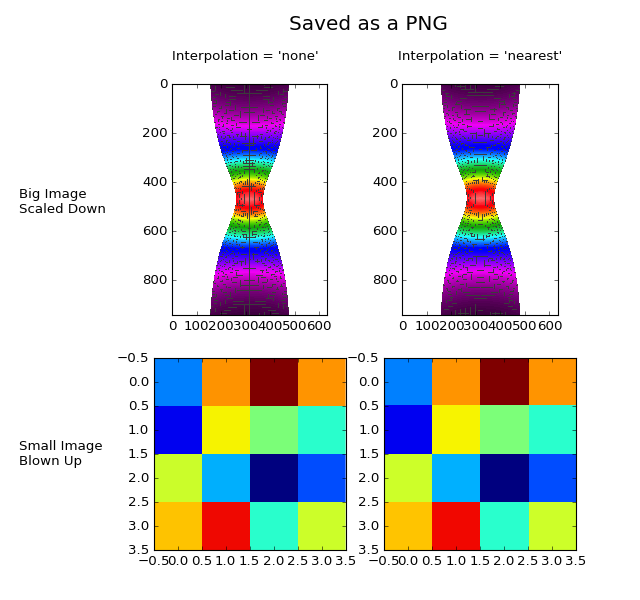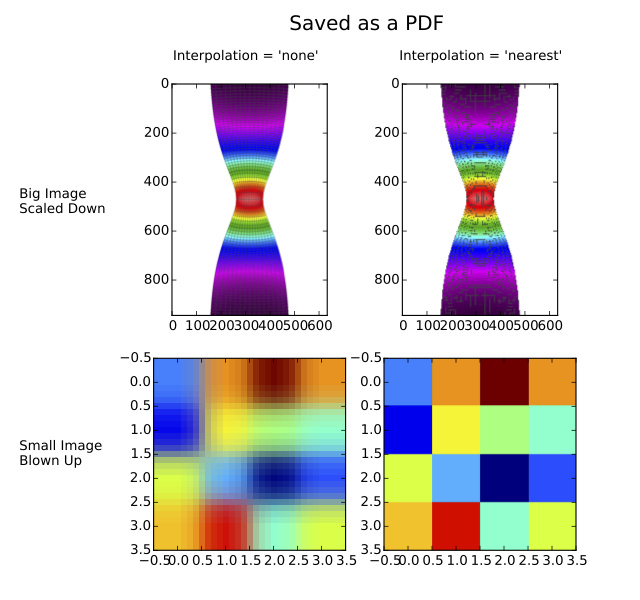## Matplotlib 2.0.0rc2 is available

Install the release candidate now!Travis-CI:# images_contours_and_fields example code: interpolation_none_vs_nearest.py¶(png, hires.png, pdf)(png, hires.png, pdf)

```"""
Displays the difference between interpolation = 'none' and
interpolation = 'nearest'.

Interpolation = 'none' and interpolation = 'nearest' are equivalent when
converting a figure to an image file, such as a PNG.
Interpolation = 'none' and interpolation = 'nearest' behave quite
differently, however, when converting a figure to a vector graphics file,
such as a PDF.  As shown, Interpolation = 'none' works well when a big
image is scaled down, while interpolation = 'nearest' works well when a
small image is blown up.
"""

import numpy as np
import matplotlib.pyplot as plt
import matplotlib.cbook as cbook

big_im_path = cbook.get_sample_data('necked_tensile_specimen.png')
# Define small image
small_im = np.array([[0.25, 0.75, 1.0, 0.75], [0.1, 0.65, 0.5, 0.4],
[0.6, 0.3, 0.0, 0.2], [0.7, 0.9, 0.4, 0.6]])

# Create a 2x2 table of plots
fig = plt.figure(figsize=[8.0, 7.5])
ax = plt.subplot(2, 2, 1)
ax.imshow(big_im, interpolation='none')
ax = plt.subplot(2, 2, 2)
ax.imshow(big_im, interpolation='nearest')
ax = plt.subplot(2, 2, 3)
ax.imshow(small_im, interpolation='none')
ax = plt.subplot(2, 2, 4)
ax.imshow(small_im, interpolation='nearest')
bottom=0.05, top=0.86)

# Label the rows and columns of the table
fig.text(0.03, 0.645, 'Big Image\nScaled Down', ha='left')
fig.text(0.03, 0.225, 'Small Image\nBlown Up', ha='left')
fig.text(0.383, 0.90, "Interpolation = 'none'", ha='center')
fig.text(0.75, 0.90, "Interpolation = 'nearest'", ha='center')

# If you were going to run this example on your local machine, you
# would save the figure as a PNG, save the same figure as a PDF, and
# then compare them.  The following code would suffice.
txt = fig.text(0.452, 0.95, 'Saved as a PNG', fontsize=18)
# plt.savefig('None_vs_nearest-png.png')
# txt.set_text('Saved as a PDF')
# plt.savefig('None_vs_nearest-pdf.pdf')

# Here, however, we need to display the PDF on a webpage, which means
# the PDF must be converted into an image.  For the purposes of this
# example, the 'Nearest_vs_none-pdf.pdf' has been pre-converted into
#'Nearest_vs_none-pdf.png' at 80 dpi.  We simply need to load and
# display it.
pdf_im_path = cbook.get_sample_data('None_vs_nearest-pdf.png')